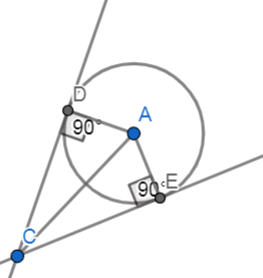Courses
Courses for Kids
Free study material
Free LIVE classes
MoreLIVE
Join Vedantu’s FREE Mastercalss

# Prove that the lengths of tangents drawn from the external point of a circle are equal.Verified
361.8k+ views
Hint: In this question, we need to draw the diagram first so it gives a clarity about what we have to find. Now, by observing the sides and angles of the triangles that we consider we need to check the congruency.

Let us look at some of the basic definitions first.

CIRCLE: Circle is defined as the locus of a point which moves in a plane such that its distance from a fixed point in that plane is constant.

TANGENT: In geometry, a tangent line to a circle is a line that touches the circle at exactly one point, never entering the circle's interior

CONGRUENCE OF TRIANGLES:

Two triangles that are identical in all respects are said to be congruent and are denoted by the symbol $\cong$. In two congruent triangles,

The corresponding sides are equal.

The corresponding angles are equal.

Conditions for two triangles to be congruent:

Three sides of one triangle are respectively equal to three sides of the second triangle (SSS).
Two sides and the included angle of one triangle respectively equal to two sides and the included angle of another triangle (SAS).

Two angles and the included side of one triangle are respectively equal to two angles and the included side of another triangle (ASA).

Two right angled triangles are congruent, if the hypotenuse and one side of one triangle are respectively equal to the hypotenuse and one side of another right angled triangle (RHS).
Now, let us draw the diagram for two tangents drawn from an external point to a circle.Let us assume a circle with centre A, CD and CE are the two tangents drawn to it from the external point C.
Required to prove that CD = CE.
So, first we need to join AD, AE, and AC.
We already know that tangent at any point of a circle is perpendicular to the radius through the point of contact.
So, we get,
\begin{align} & CD\bot AD \\ & CE\bot AE \\ \end{align}
Now, by considering the triangles ACD and ACE we get,
$\angle ACD=\angle ACE$
AC = AC (common side for both the triangles)
Hence , by RHS congruence as mentioned above we can say that,
$\vartriangle ACD\cong \vartriangle ACE$
As both the triangles are congruent. So, from the properties of congruent triangles:
$\therefore CD=CE$
Thus, the length of two tangents drawn from an external point to a circle are equal.

Note: It is important to note that the tangent at any point of a circle is perpendicular to the radius through the point of contact.
We need to be careful while observing the angles and the sides in order to prove the congruence. So, that the condition of congruency we use changes accordingly.
For example, when we consider the triangles ACE and ACD establishing that
$\angle ACD=\angle ACE$
Is one of the crucial steps here because we can consider it from the above relation we got.
\begin{align} & CD\bot AD \\ & CE\bot AE \\ \end{align}.

Last updated date: 26th Sep 2023
Total views: 361.8k
Views today: 8.61k